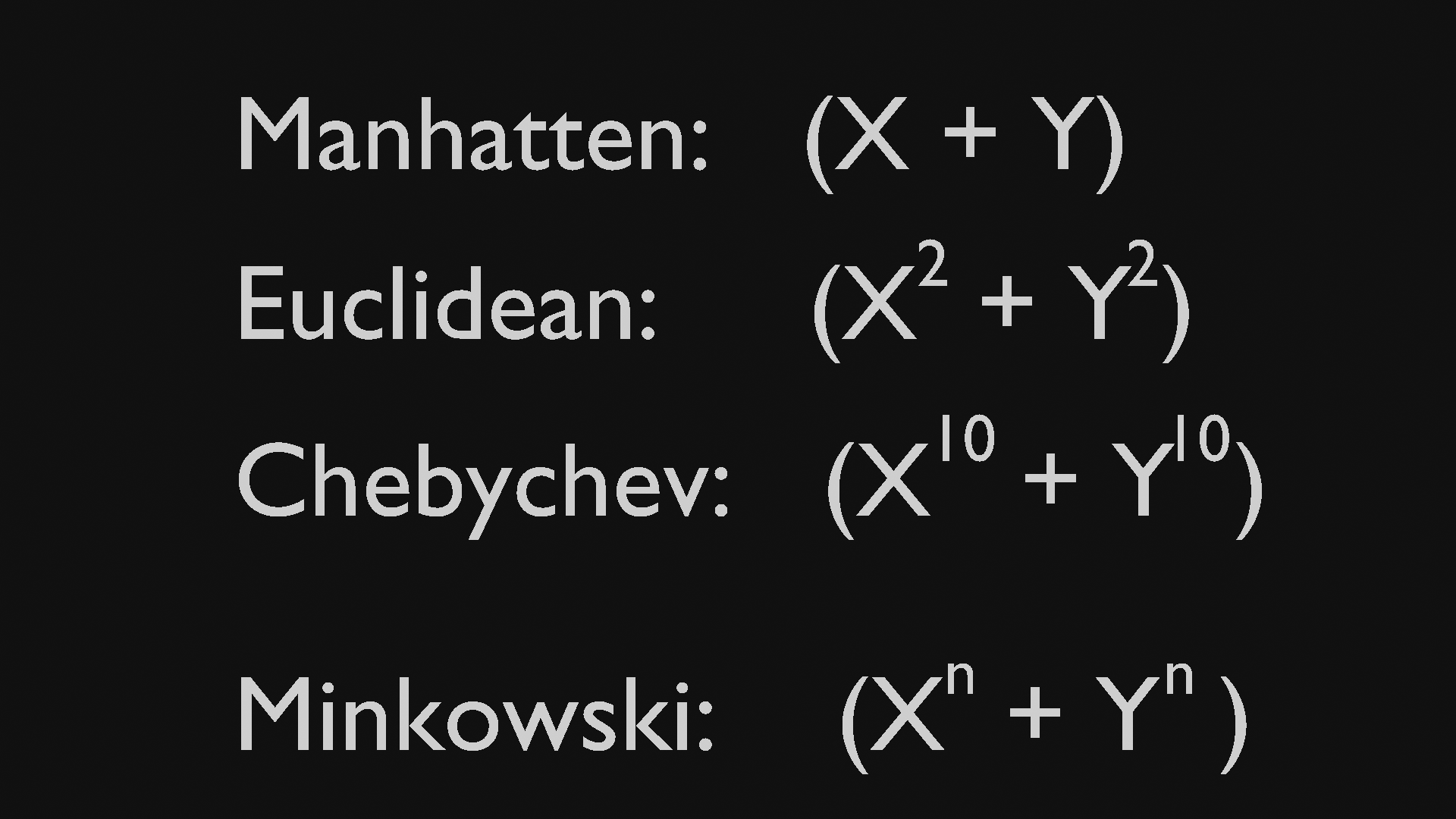2 answers · asked · Lesson: Intro to Procedural Texturing · Course: Fundamentals of Texturing in Blender

Distances in Voronoi

In a nutshell:

The 4 different methods to calculate the distance between two points are:where X stands for the (absolute) difference in the x coordinates and Y for the (absolute) difference between the y coordinates. And the n in the Minkowski distance is the exponent in Blender.

F1 calculates the distance between two neighboring points and F2 between one point and the one 'next to the neighbor'...

• Erratum:

The correct form of  the Minkowski distance should (of course) be: the n-th root of the term between brackets!

And accordingly the other distances (the Euclidean distance is the square root of (X² + Y²), and so on...).

This logically extends to 3 dimensional distances as you would expect (the square root of (X² + Y² + Z²), etc.)

This is only important if you actually want to calculate those distances; the maiin thing I wanted to show is, that those mysterious names that 'no-one' can pronounce, just mean various ways to calculate distances  and that there is a simple connection between them...which the above image shows pretty well, I think, although technically not correct...

• Great note for those curious!